# fairmodels## Overview

Flexible tool for bias detection, visualization, and mitigation. Use models explained with DALEX and calculate fairness classification metrics based on confusion matrices using `fairness_check()` or try newly developed module for regression models using `fairness_check_regression()`. R package fairmodels allows to compare and gain information about various machine learning models. Mitigate bias with various pre-processing and post-processing techniques. Make sure your models are classifying protected groups similarly.

## Installation

Install it from CRAN:

``install.packages("fairmodels")``

or developer version from GitHub:

``devtools::install_github("ModelOriented/fairmodels")``

## Example

Checking fairness is easy!

``````library(fairmodels)
library(ranger)
library(DALEX)

data("german")

# ------------ step 1 - create model(s)  -----------------

lm_model <- glm(Risk~.,
data = german,

rf_model <- ranger(Risk ~.,
data = german,
probability = TRUE,
num.trees = 200)

# ------------  step 2 - create explainer(s)  ------------

# numeric y for explain function
y_numeric <- as.numeric(german\$Risk) -1

explainer_lm <- explain(lm_model, data = german[,-1], y = y_numeric)
explainer_rf <- explain(rf_model, data = german[,-1], y = y_numeric)

# ------------  step 3 - fairness check  -----------------

fobject <- fairness_check(explainer_lm, explainer_rf,
protected = german\$Sex,
privileged = "male")

print(fobject)
plot(fobject)
``````

Compas recidivism data use case: Basic tutorial

## How to evaluate fairness in classification models?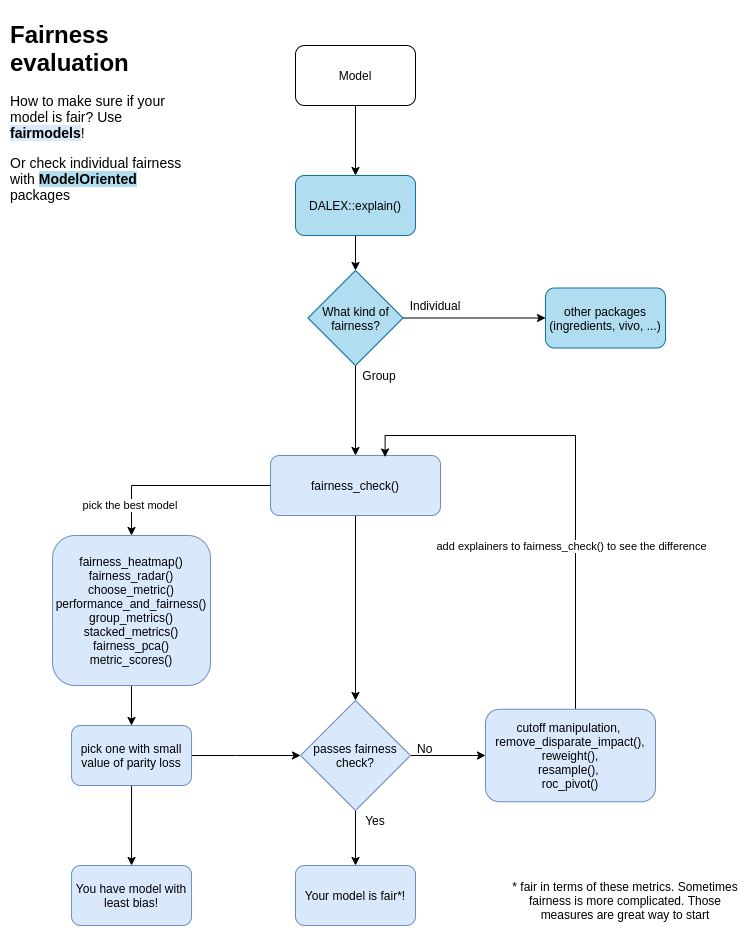### Fairness checking is flexible

`fairness_check` parameters are
* x, … - `explainers` and `fairness_objects` (products of fairness_check).
* protected - factor with different subgroups as levels. Usually specific race, sex etc…
* privileged - subgroup, base on which to calculate parity loss metrics.
* cutoff - custom cutoff, might be single value - cutoff same for all subgroups or vector - for each subgroup individually. Affecting only explainers.
* label - character vector for every explainer.

Models might be trained on different data, even without protected variable. May have different cutoffs which gives different values of metrics. `fairness_check()` is place where `explainers` and `fairness_objects` are checked for compatibility and then glued together.
So it is possible to to something like this:

``````fairness_object <- fairness_check(explainer1, explainer2, ...)
fairness_object <- fairness_check(explainer3, explainer4, fairness_object, ...)``````

even with more `fairness_objects`!

If one is even more keen to know how `fairmodels` works and what are relations between objects, please look at this diagram class diagram

## Metrics used

There are 12 metrics based on confusion matrix :

Metric Formula Full name fairness names while checking among subgroups
TPR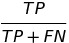true positive rate equal opportunity
TNR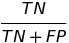true negative rate
PPV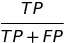positive predictive value predictive parity
NPV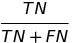negative predictive value
FNR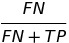false negative rate
FPR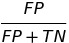false positive rate predictive equality
FDR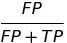false discovery rate
FOR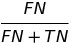false omission rate
TS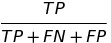threat score
STP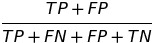statistical parity statistical parity
ACC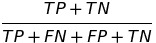accuracy Overall accuracy equality
F1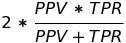F1 score

and their parity loss.
How is parity loss calculated?

Where `i` denotes the membership to unique subgroup from protected variable. Unprivileged subgroups are represented by small letters and privileged by simply “privileged”.

some fairness metrics like Equalized odds are satisfied if parity loss in both TPR and FPR is low

#### How easy it is to add custom fairness metric?

It is relatively easy! Check it out here

### Fairness in regression

R package fairmodels has support for regression models. Check fairness using `fairness_check_regression()` to approximate classification fairness metrics in regression setting. Plot object with `plot()` to visualize fairness check or with `plot_density()` to see model’s output.

Zafar, Valera, Rodriguez, Gummadi (2017) https://arxiv.org/pdf/1610.08452.pdf

Barocas, Hardt, Narayanan (2019) https://fairmlbook.org/

Steinberg, Daniel & Reid, Alistair & O’Callaghan, Simon. (2020). Fairness Measures for Regression via Probabilistic Classification. - https://arxiv.org/pdf/2001.06089.pdf